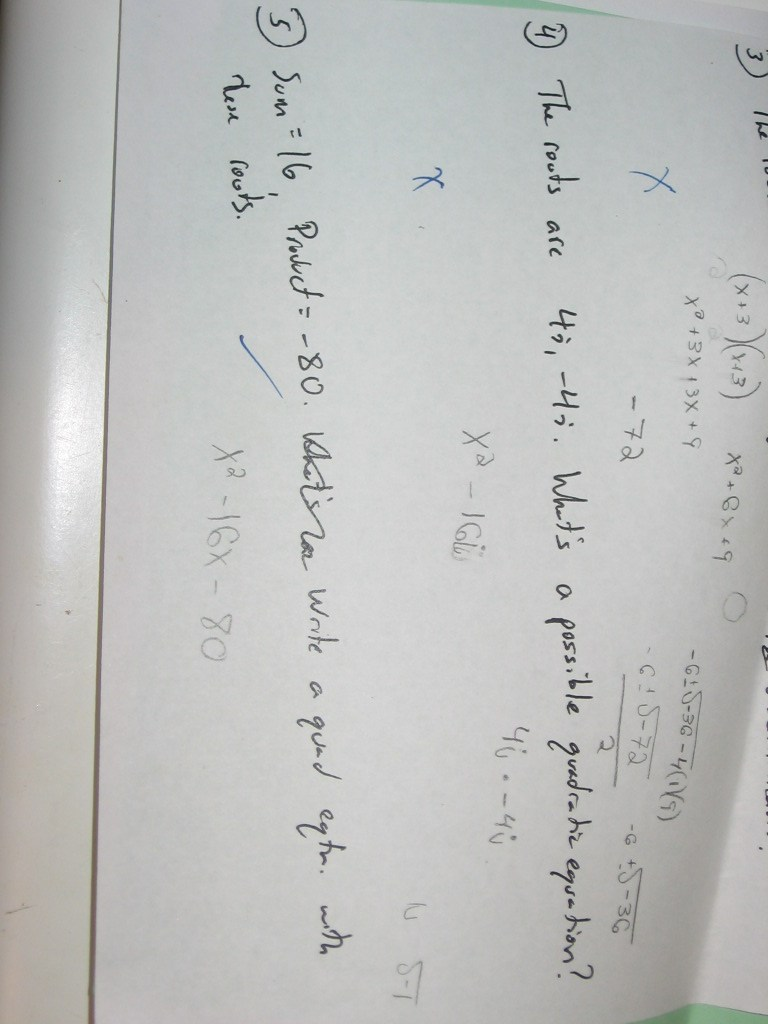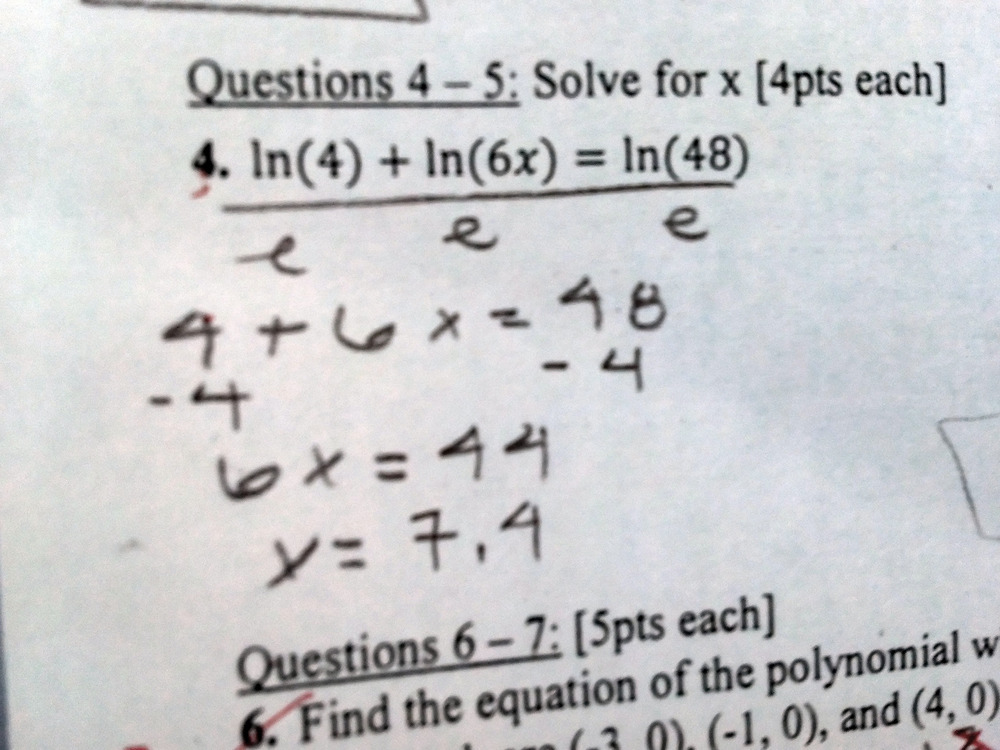Categories

## From complex roots to a quadratic equationHow did this student end up with the answer he did?  What does it seem that he knows about complex numbers?

Categories

## Adding Complex NumbersWhat is it about complex numbers that this student finds confusing? Do you think he would make an identical mistake on$(12 + 3x) + (2 - 3i)$? Why or why not?

Categories

## Keeping your composure (? does that even count as a pun?)What does this student know about composition of functions?

What’s going through the kid’s brain when he makes his mistake?

How would you help?

Categories

## Equation of ParabolaFrom the student’s work, what can we infer that the student knows?  What is the student thinking? How would you help?

Categories

## Dividing by “log”Last week we posted a somewhat similar mistake involving logarithms. Here’s the student work:While you should feel free to comment on today’s mistake independent of the earlier one, I think it would be especially productive to compare these two pieces of student work. Could the same student have done both pieces of work? Are there differences between the way each student understands logarithms?

By the way, we now support equation editing in LaTeX, so feel free to write things such as$\frac{2\log(6x)}{\log}$ in the comments. This post is also tagged with a Common Core Standard and categorized according the hierarchy that you see in the menu on the left.

CategoriesToday’s mistake is a classic, at least in my classroom.  What makes this mistake so tempting for students, and how do you help them see the light?

Categories

## Dividing by “e”

Why did this student divide by “e”? What does that say about the way they think about logarithms? How would you help the student?

Categories

## Composition of Functions

This kid clearly knows some of the basics of composing functions. But check out that last line.

What is going through this kid’s brain, and how would you respond?

Categories

## Trigonometry – Find the missing angle

My sense is that this mistake isn’t as interesting as the rest, but it’s a pretty common one that I see in Trigonometry. The question is, what sort of activity would help this student out?

Categories

## Not-so-simple simplifying

What’s the student thinking, and how can we help?